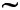# Similar Figures Test

Name:______________________________________

Date:_____________________Referring to the above figure, answer the following:

1. What is the value ofKML? (click for answer)

2. What is the value ofPRQ? (click for answer)

3. What is the length of PQ? (click for answer)

4. Can we sayKLMPQR? (click for answer)

As,KLM =PQR,KML =QRP andLKM =QPR

KL = KM = LM
PQ PR QR